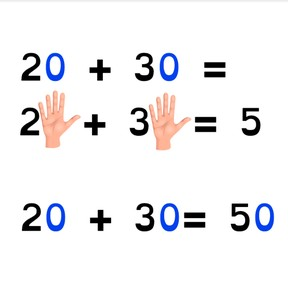Addition to 100 with the zero-rule

# Addition to 100 with the zero-rule

No account needed.8,000 schools use Gynzy92,000 teachers use Gynzy1,600,000 students use Gynzy

## General

Students can add tens numbers to 100 using the zero rule. They learn to add tens quickly by removing the zero to add the zero to the answer.

2.NBT.B.7
2.OA.A.1

## Relevance

If students are able to quickly add to 100 using the zero rule, they can quickly add tens numbers.

## Introduction

Using a ball, practice adding numbers to 10 quickly. You say an addition problem (max 10) and throw the ball to a student. They must answer and throw the ball back. Repeat a few times.

## Development

Discuss with students that it is important to use the zero rule to make adding tens numbers easier. Next, discuss that tens numbers always end on a 0, and name all the tens to 100. Show how the zero rule works by showing 1+1 and 10+10 with fingers, fists and the MAB blocks, so students have a visual support to their understanding of the zero rule.
Next, explain the zero rule using an example. Show that you can park the zeros in an addition problem with tens and solve the easier problem first. You make the addition problem ten times smaller by parking the zero. To solve, you replace the zeros, but then you replace the zero after the answer too. Practice this with the students with the three exercises given. Make sure that they park or remove the zero to quickly solve, and then return the zero to the problem and answer.

Check that students are able to add to 100 using the zero rule by asking the following questions:
- Which numbers do you count with the zero rule?
- What do you do with tens if you want to add easily and quickly?
- What do you do with the answer if there are zeros in both addends?

## Guided practice

Students first practice with an exercise where the total without zero is already given, and next they must determine the total of the problem without zeros as well as with zeros.

## Closing

Check that students can add tens using the zero rule by asking what to do with tens if you want to add easily and quickly. Ask students why it is useful to be able to do this and ask what 30+50 is. Next, students can show addition problems to 100 with their hands. Have them first create a problem without zeros and then calculate what the total with zeros would be.

## Teaching tips

If students have difficulty adding to 100 using the zero rule, you can practice a few more problems with them together. Make sure that students understand the steps of parking the zero, solving and then replacing he zeros. Start with smaller problems like 20+10 and slowly grow to problems like 70+30.

## Instruction materials

A ball

### The online teaching platform for interactive whiteboards and displays in schools

• Save time building lessons

• Manage the classroom more efficiently

• Increase student engagement